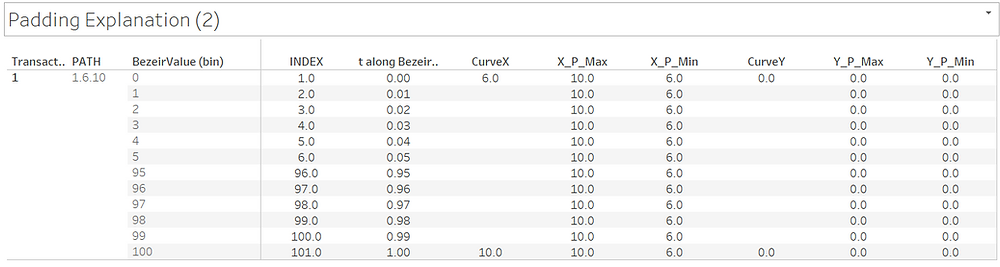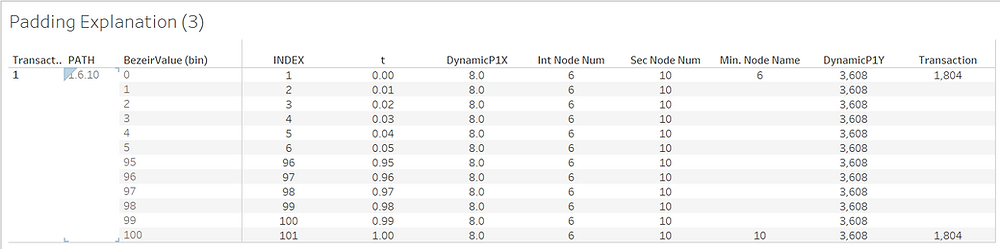# Tableau Note: How To Create the Jump Plot.

About the Jump Plot a reference is here. I recommend you to look through it to have a big picture. I also published my Tableau Workbook.

As a short explanation of the Jump Plot, it shows a sequence with a measure shown as a hopping curve. It could help you see how the sequence is, if there's a bottleneck or an outlier and so on.

I created dummy data with the R codes below. If you don't use R, you can download the data here.The data looks like this. It has transaction ID, Date of when the transaction is done, Who made it to whom and the amount of the transaction. (I realized that The date should have been sorted first to match it with the Transaction ID in order to make it more realistic. Sorry if you feel uncomfortable with the data.)

By the way, the last time I made the Network Graph, I used the data aggregated in stead of the original data, which decreases Tableau's interactability because you can not filter the data as you want. This time I only use the original data and I will revise the article later.

The first thing is to union the same original data.Then Let's make some calculations.

Path Order

IIF([Table Name]='original.csv',1,2)

Node Name

IIF([Path Order]=1,[Name_from],[Name_to])

PATH

STR([Transaction_ID])+'.'+STR([Name_from])+'.'+STR([Name_to])

BezeirValue

IIF([Path Order]=1,0,100)

And We need BezeirValue(bin) where the bin size is 1. This is for data padding. If you are not familiar with this idea, this workbook-Viola Chart and Data Padding could help you out.

PATH works as a unique Identifier of each record (well, actually it's enough to use only the Transaction ID because it's unique for the each record.)

Then we create two INDEX calculations.

INDEX

INDEX()

t

([INDEX]-1)/100

Now we are using Table Calculation and all of the Table Calculations should have one strict order about what is used for the calculations and where to restart them.Open the Calculation Edit Window and Click "Default Table Calculation", then config it like this. Notice that Addressing Order is important. PATH is first, then BezeirValue(bin). And set PATH in "Restarting every".Again, all of the calculations using Table Calculation should be set in this way. If you don't get the same result with the Jump Plot, you may have made a mistake with this.

And we also pad Transaction to give each INDEX(each point for the line) the Transaction. I will explain later so let me show the calculation.

WINDOW_MIN(MIN([Transaction]),FIRST(),FIRST())

Actually It doesn't matter if you use MIN, MAX or AVG. To explain it, let's see how these Table Calculations work.I extracted only one PATH. Each PATH has BezeirValue(bin) from 1 to 100, but Transaction has the value only in 0 and 100 where Path Order has a value, which means that between 1 to 100 all points don't have a measure to be used to create the curve. Then WINDOW function is used to duplicate and address the Transaction on each points.

(By the way if you don't see the bin has 1 to 100 continuously, you have to make it show the missing values. Please don't forget it.)

So, this is the preparation for the calculations format the Jump Plot.

The first step is assign X-value and Y-Value on the checkpoints. They are named as CurveX/Y but I don't know why.

CurveX

[Node Name]

CurveY

0

So basically CurveX gives you where the check points are, and at the check points Y-value should be 0 because the curve in the Jump Plot should come back to the check points.

Then we calculate these below.

X_P_Max

WINDOW_MAX(AVG([CurveX]))

X_P_Min

WINDOW_MIN(AVG([CurveX]))

Y_P_Max

WINDOW_MAX(AVG([CurveY]))

Y_P_Min

WINDOW_MIN(AVG([CurveY]))My understanding on these calculation is that basically they give each points the information of where to start and where to end. X_P_Min shows where to start and X_P_Max shows where to ends. (I feel Y_P_Max/Min is for an application but in this case they are just zero.)

Then we calculate these below.

Int Node Num

WINDOW_MIN(MIN([Node Name]),FIRST(),FIRST())

Sec Node Num

WINDOW_MIN(MIN([Node Name]),LAST(),LAST())

DynamicP1X

WINDOW_MIN(FLOAT([Int Node Num]) + ((FLOAT([Sec Node Num])-FLOAT([Int Node Num]))/2))

DynamicP1YBasically Dynamic P1Y gives you where the top of the curve will be. Int/Sec Node Num seem to be just data padding of where to start and where to end. As for the DynamicP1Y, I don't know why it's doubled, but basically it gives you the y-value at the top of the curve.Then, now finally we can create the core component.

BezierX

(1-[t])^2*[X_P_Min])+(2*(1-[t])*[t]*[DynamicP1X])+(([t]^2)*[X_P_Max])

BezierY

((1-[t])^2*[Y_P_Max])+(2*(1-[t])*[t]*[DynamicP1Y])+(([t]^2)*[Y_P_Min])

Well, I put a reference for the Bezier Curve, but honestly I don't know what it is. Let me just focus on how to create the Jump Plot.

Then you can arrange components like this.

The video below could be helpful to understand how data padding helps us create the curve.

And you can also download my Tableau Public Workbook to understand what these processes are.

When I met the Jump Plot for the first time, I couldn't understand how to create it. It took me days to analyze it, so I hope this article could be helpful for you.

In the workbook I added the Jump Plot with aggregated measures too.